## ThinkDSP¶

This notebook contains code examples from Chapter 11: Modulation and sampling

Special thanks to my colleague Siddhartan Govindasamy; the sequence of topics in this notebook is based on material he developed for Signals and Systems at Olin College, which he and Oscar Mur-Miranda and I co-taught in Spring 2015.

In :
# Get thinkdsp.py

import os

if not os.path.exists('thinkdsp.py'):
!wget https://github.com/AllenDowney/ThinkDSP/raw/master/code/thinkdsp.py

In :
import numpy as np
import matplotlib.pyplot as plt

from thinkdsp import decorate


## Convolution with impulses¶

To demonstrate the effect of convolution with impulses, I'll load a short beep sound.

In :
if not os.path.exists('253887__themusicalnomad__positive-beeps.wav'):

In :
from thinkdsp import read_wave

wave.normalize()
wave.plot()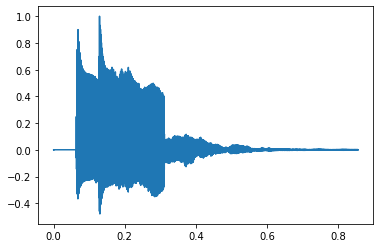Here's what it sounds like.

In :
wave.make_audio()

Out:

And here's a sequence of 4 impulses with diminishing amplitudes:

In :
from thinkdsp import Impulses

imp_sig = Impulses([0.005, 0.3, 0.6, 0.9], amps=[1, 0.5, 0.25, 0.1])
impulses = imp_sig.make_wave(start=0, duration=1.0, framerate=wave.framerate)
impulses.plot()
decorate(xlabel='Time (s)')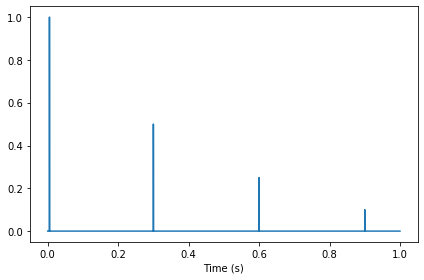If we convolve the wave with the impulses, we get 4 shifted, scaled copies of the original sound.

In :
convolved = wave.convolve(impulses)
convolved.plot()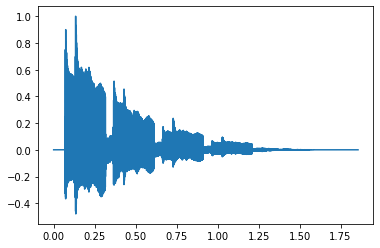And here's what it sounds like.

In :
convolved.make_audio()

Out:

## Amplitude modulation (AM)¶

The previous example gives some insight into how AM works.

In :
if not os.path.exists('105977__wcfl10__favorite-station.wav'):
!wget https://github.com/AllenDowney/ThinkDSP/raw/master/code/105977__wcfl10__favorite-station.wav

In :
wave = read_wave('105977__wcfl10__favorite-station.wav')
wave.unbias()
wave.normalize()
wave.make_audio()

Out:

Here's what the spectrum looks like:

In :
spectrum = wave.make_spectrum()
spectrum.plot()
decorate(xlabel='Frequency (Hz)')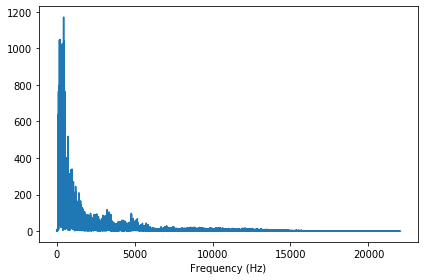For the following examples, it will be more useful to look at the full spectrum, which includes the negative frequencies. Since we are starting with a real signal, the spectrum is always symmetric.

In :
spectrum = wave.make_spectrum(full=True)
spectrum.plot()
decorate(xlabel='Frequency (Hz)')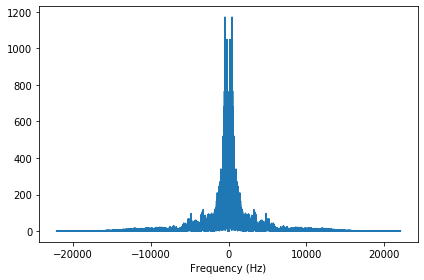Amplitude modulation works my multiplying the input signal by a "carrier wave", which is a cosine, 10 kHz in this example.

In :
from thinkdsp import CosSignal

carrier_sig = CosSignal(freq=10000)
carrier_wave = carrier_sig.make_wave(duration=wave.duration, framerate=wave.framerate)


The * operator performs elementwise multiplication.

In :
modulated = wave * carrier_wave


The result sounds pretty horrible.

In :
modulated.make_audio()

Out: